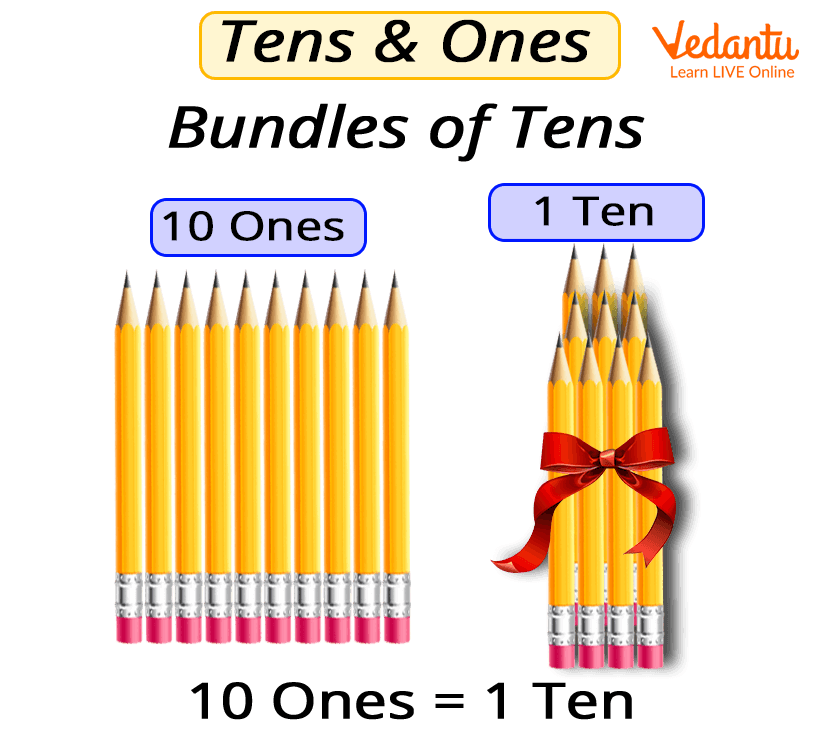Courses
Courses for Kids
Free study material
Free LIVE classes
More

# The Concept of Place Value in Grade 1## Introducing Place Value to Kids

Have you ever wondered how we define the value of a digit in large numbers like 100, 123, or 78? Well, we do that by assigning them a place value. The place value and the use of the place value chart is one of the essential concepts taught to kids in Grade 1. Some of the basic concepts that students are taught include the meaning of place value and determining the place value. So, here we have attempted to provide a concise description of the concept. We have also provided some of the most efficient tips to introduce the concepts to the kids.

## What Does Place Value Mean?

The concept of place value is quite useful to us. It allows us to arrange the given digits in a number as per the position. For example, Riya likes the 23 and 45 numbers a lot and she wants to know the position of each digit in this number, so she finds that 5 is at the right most position and this position is the unit’s or one’s place. Similarly, 2 is at the left most position, which is called the tens place.

A question arises how do we determine the position of 2, 3, and 4, 5 in a given number set. Now, for the kid at the age of 5-6, understanding the place value in Year 1 becomes essential in order to determine this.

So, how do we make the kid understand the place value? Let us go through this article to understand the place value for a Grade 1 kid.

## How Grade 1 Kids Can Be Taught the Place Value of Digits?The concept of place value in Grade 1

For this, a child in Grade 1 starts to learn to count up to and somewhat beyond 100 in multiples of 1, 2, 5, and 10. They also count aloud and represent numbers using pictures and concrete objects like sticks, straws, number lines, and so on.

So, the student understands the concept of place value via visual representation, this is what we will talk about.

## Tips to Help Students in Grade 1 to Understand Place Value

• Talk about Numbers Around Him/Her

Everywhere we can find numbers and these numbers can tell us all sorts of different things. For example, some numbers tell us about the number of pens in a packet, the amount of something in a container or jar (for example, ‘there are ten sweets in the jar’).

Some tell us about your sibling’s position in a race (for example, ‘He finished the race in 1st place’). And some are just used for labelling (for example, ‘we are waiting for the train number 3307’).

Talking about how we use numbers in the real world can help a student understand why they are important. For example, you could talk about numbers when you are going to some distance, buying something, telling the time, or catching a train at a particular time.

• Count

A Year 1 student should know why, how, and when to count.

You can bring this into his/her practice by counting orally through rhymes or songs and or make any short poem like ‘Ten Green Bottles’, going forwards and backward. Have a go with our fun song:

Counting objects surrounding your students is also a good way to make sure him/her understands that each number name can portray an amount. Try counting in steps. For example, you can count pieces of chocolates in 5s, use your fingers to count in fives, or count 10 pieces in one chocolate.

• Make Representations of Numbers

The significant factor in understanding the place value is that your students understand what numbers are, how they look at them, so it is helpful to represent numbers using images of objects around you.

For example, to understand the number 5, children should know what 5 really is. We can represent it by drawing the symbol, 5 on the whiteboard. However, it is also important to make him/her understand what 5 looks like as a quantity. You can make five sticks on the whiteboard, count those to show ‘5’.

## Conclusion

From the above text, we understand that for a child learning the concept of place value in Grade 1, they need to understand that numbers can be made up of other numbers. So two-digit numbers can be made of two ones, or a two and a one. Once they reach the teen numbers, we should start showing them that these are numbers made from one ten, plus several ones. For example, nineteen is made of one ten and nine ones. Again, this is much easier to explain to your students using objects or pictures of the same.

Last updated date: 21st Sep 2023
Total views: 129.9k
Views today: 3.29k

## FAQs on The Concept of Place Value in Grade 1

1. What is the place value of 3 in the number 34?

34 can be written as

4 is at  the one’s place, so 4 * 1 = 4

Similarly, 3 is at the left position so we add 0 after 1 and get 10. Now, multiplying it by 3, we get 3 * 10 = 30

Here, 0 after 3 makes it 30, which is actually the ten’s position of 3 in a number 34.

2. What concepts of place value can be taught to students of Grade1?

Tens and Ones in a given number are called place values. Each digit in a number has a position. So, place values tell us how much each digit in a number is worth, where digits on the left have a bigger place value and the place value keeps decreasing while proceeding to the right.